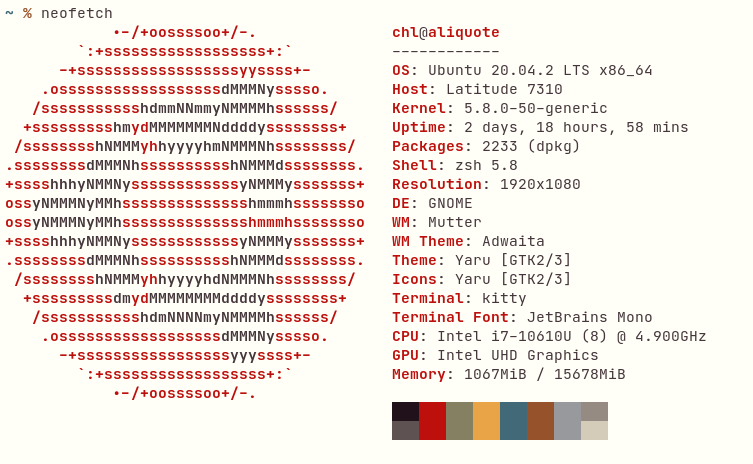# aliquote

## < a quantity that can be divided into another a whole number of time />

My color scheme for the terminal is rather unusual, but it is in part inspired by the Belafonte Day color theme. I initially configured Kitty to use my custom theme, and then extend it to other terminal emulators and text editors, although for the later I don’t really use colors (except for highlighting strings, number and all sorts of literals). Actually, I’m only using 10 colors instead of 16, meaning I use the same low and high contrast color for all colors except black and white – which are not really black and white, by the way.In case I need it, it would be nice to be able to slightly decrease the luminance of the alternative colors. Since we are working using Hex codes, it involves a bit more work than with HSL. In essence, this has been covered in this blog post, or in various StackOverflow threads.

I originally thought that this would be a perfect task for a foldr-like recursion in Racket (or Haskell). However, a simpler solution would be to rely on Racket’s for/fold form which facilitates the use of accumulators. Converting an Hex number to its decimal counterpart lends itself well to recursive writing: it is enough to iterate over each digit, from right to left, and add them up once weighted by 16 times their previous weight, where for the base case the weight equals 1. As for the guard of this recursive solution, we just have to check that there are no more digit to proceed with. This approach is known as Horner’s rule, which is widely used in the case of polynomials. Indeed, for any base $b$, the decimal representation of a number can be found from the following expansion:

$$\dots + x_2b^2 + x_1b^1 + x_0b^0 + x_{-1}b^{-1} + x_{-2}b^{-2} + \dots,$$

As we did earlier, to convert a number in Hex notation to its decimal representation we can use number->string directly, as in (number->string #x7e5 10) (which should return “2021”). In the above polynomial expansion, negative exponents and indices represent the fractional parts of the number. If you only work with integers, you can safely remove everything after $x_0b^0$. As an example, consider the base 2 number 11012:

\begin{align} 1101_2 & = (1 \cdot 2^3 + 1 \cdot 2^2 + 0 \cdot 2^1 + 1 \cdot 2^0)_{10} \cr & = (((0 \cdot 2 + 1) \cdot 2 + 1) \cdot 2 + 0) \cdot 2 + 1 \cr & = 13_{10} \end{align}

Same idea for the Hex number above (without assuming that #7E5 expands to #77EE55):

\begin{align} \text{#7E5} & = (7 \cdot 16^2 + 14 \cdot 16^1 + 5 \cdot 16^0)_{10} \cr & = 2021_{10} \end{align}